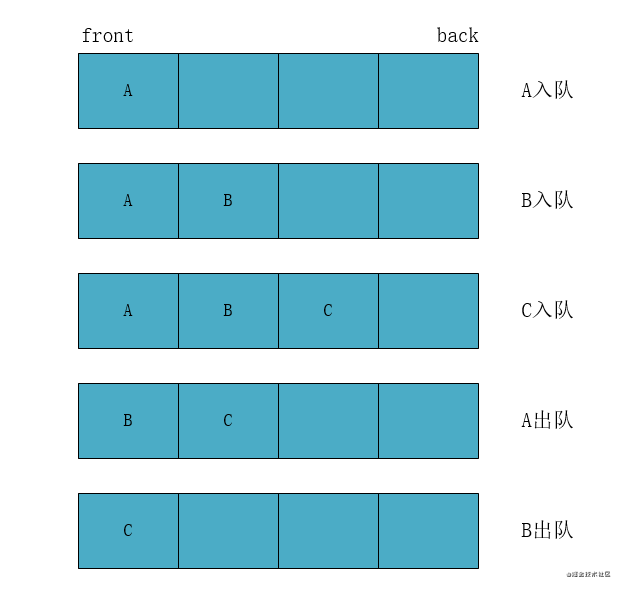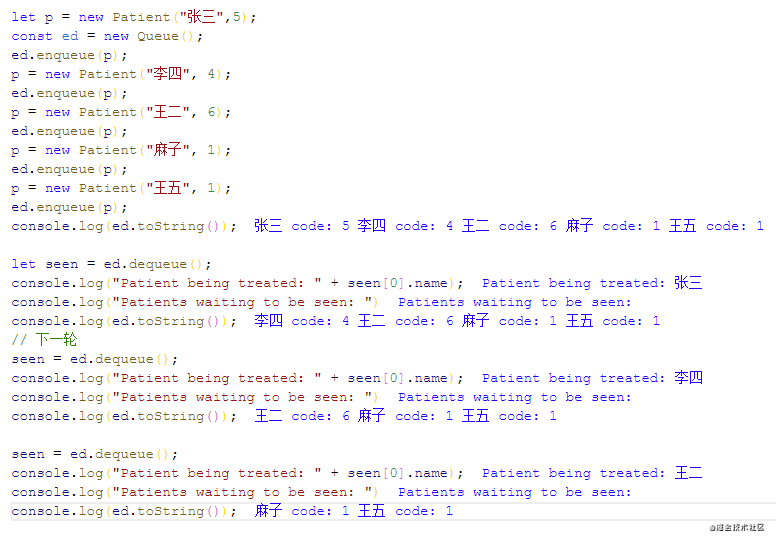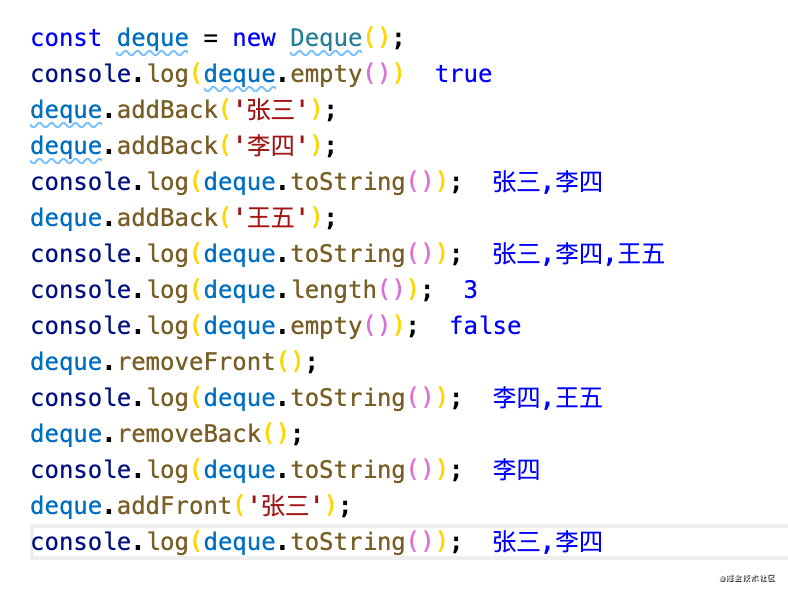# 基础队列## 构建Queue类

``````class Queue {
constructor() {
this.dataStore = [];
}
enqueue(element) {
this.dataStore.push(element);
}
dequeue() {
return this.dataStore.shift();
}
front() {
return this.dataStore;
}
back() {
return this.dataStore[this.dataStore.length - 1];
}
empty() {
return this.dataStore.length === 0;
}
toString() {
return this.dataStore.toString();
}
length() {
return this.dataStore.length;
}
}
``````

# 优先队列

``````class Patient {
constructor(name, code) {
this.name = name;
this.code = code;
}
}
``````

``````dequeue() {
let priority = this.dataStore;
for (const i = 0, len = this.dataStore.length; i < len; i++){
if (this.dataStore[i].code < priority) {
priority = i;
}
}
return this.dataStore.splice(priority, 1);
}
``````

``````toString() {
let retStr = "";
for (var i = 0; i < this.dataStore.length; ++i) {
retStr += `\${this.dataStore[i].name} code: \${this.dataStore[i].code}\n`;
}
return retStr;
}
``````# 双端队列

## 创建Deque类

``````class Deque {
constructor() {
this.dataStore = [];
}
if(this.empty()){
}else{
this.dataStore.unshift(element);
}
}
this.dataStore.push(element);
}
removeFront() {
return this.dataStore.shift();
}
removeBack() {
return this.dataStore.pop();
}
front() {
return this.dataStore;
}
back() {
return this.dataStore[this.dataStore.length - 1];
}
empty() {
return this.dataStore.length === 0;
}
toString() {
return this.dataStore.toString();
}
length() {
return this.dataStore.length;
}
}
``````

## 测试Deque类的代码## 实际应用–>回文字符串的判断

``````function isPalindrome(word) {
if (typeof word !== "string") {
throw TypeError(`参数不是string类型`);
}
let tmp = new Deque();
for (let element of word) {
}
while (tmp.length() > 1) {
if (tmp.removeFront() !== tmp.removeBack()) {
return false;
}
}
return true;
}

console.log(isPalindrome("racecar")) // true
console.log(isPalindrome("hello")) // false
``````

# 参考资料

• 数据结构与算法JavaScript描述
• 学习JavaScript数据结构与算法 第3版